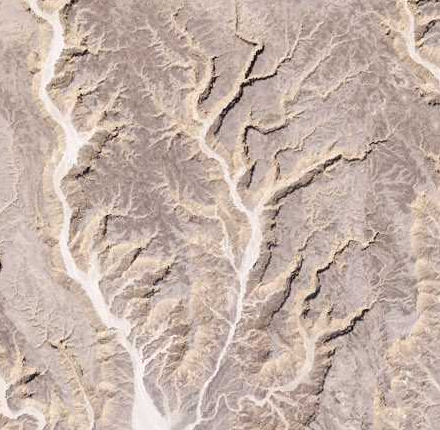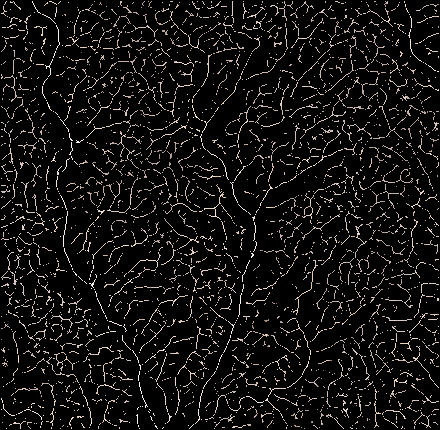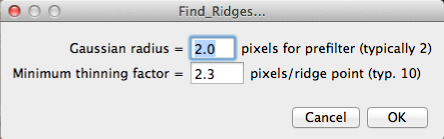Find Ridges

Author: Bob Dougherty
Description: Plugin to find the ridge points of the grey value in an image. Pixels corresponding to ridge points are not changed. Pixels that are not identified as ridge points are set to 0. There are two dialog input: "Gaussian radius" and "Minimum thinning factor." The Gaussian radius should be chosen to be comparable to the pixel width of the ridges to be found. Features that are much narrower or much wider than this value will not be identified as ridges. A typical value is 2 pixels. The "Minimum thinning factor" is the minimum factor by which the number of ridge points is smaller than the total number of pixels. For example, if the image has 512 x 512 = 262144 pixels, and "Minimum thinning factor" is given as 10, then the maximum number of pixels that will not be set to zero by the plugin is 26214. If the number of ridge points identified by the algorithm is less than 26214, then the "Minimum thinning factor" will have no effect. If a greater number of ridge points is initially identified, then some of them will be disallowed, to bring the total down to 26214. The ridge points that are retained are the ones that correspond to the strongest ridges in the sense of having the most negative second derivative, divided by the pixel value, in the direction perpendicular to the ridge.Detail of the Nile Bend sample image before and after finding ridges.Dialog.
History: April 20, 2014 initial version.
May 2, 2014 improved accuracy